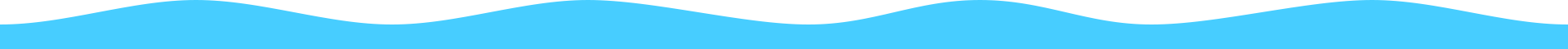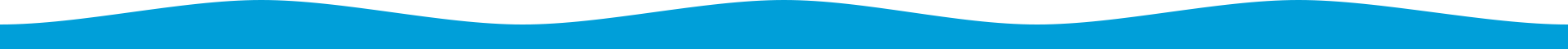• 服务对比
• 无忧服务(5分钟响应)
• 企业服务(3分钟响应)
• VIP服务(1分钟响应)

6年

3万+

2万+

• 无忧服务（免费）
• 企业服务（咨询客服）
• VIP服务（咨询客服）
• Raid组建配置
• ARP防御支持
• 数据库安装配置
• WEB环境
• 网站环境故障排查
• 破解密码
• 流量监控
• 系统安全加固
• 网站篡改通知处理
• 操作系统安装（不限次数）
• 备机备件
• 流量清洗
• WAF
• 服务器监控报表
• 模块光纤备件
• 机房驻地办公位
• ......
• Raid组建配置
• ARP防御支持
• 数据库安装配置
• WEB环境
• 网站环境故障排查
• 破解密码
• 流量监控
• 系统安全加固
• 网站篡改通知处理
• 操作系统安装（不限次数）
• 备机备件
• 流量清洗( 3G )
• WAF( 5个域名 )
• 服务器监控报表
• 模块光纤备件
• 机房驻地办公位
• ......
• Raid组建配置
• ARP防御支持
• 数据库安装配置
• WEB环境
• 网站环境故障排查
• 破解密码
• 流量监控
• 系统安全加固
• 网站篡改通知处理
• 操作系统安装( 不限次 )
• 备机备件
• 流量清洗( 5G )
• WAF( 10个域名 )
• 服务器监控报表
• 模块光纤备件
• 机房驻地办公位
• ......

Raid组建配置

RAID(磁盘阵列)安装配置，

• 售前支持
• 安装调试
• 技术支持
• 安全服务
• 服务支持
• 需求分析
• 方案设计
• 快递代收（用户付费）
• 一对一用户经理
• 上门取设备
• 硬件验收
• 加电测试
• Raid组建配置
• 操作系统安装（3次/年）
• 服务器上架
• 综合布线
• 网络设备配置
• 成品六类千兆网线
• 堡垒机配置
• 硬件VPN配置
• 查看系统状态
• 重启
• 断网/联网/开机/关机
• WEB环境
• 数据库安装配置
• 软件安装配置
• 硬件故障排查
• 软件故障排查
• 网站环境故障排查
• 远程不通
• 破解密码
• 查杀病毒
• 物理链路故障排查
• 流量监控
• 存活监控
• 性能监控
• 异常告警
• 数据备份
• 备机备件
• windows备份还原
• 模块光纤备件
• 服务器监控报表
• 网络架构分析优化
• 漏洞病毒预警
• ARP防御支持
• 系统安全加固
• 僵尸木马蠕虫通报处理
• 网站篡改通知处理
• 流量清洗
• WAF
• 安全渗透测试
• 域名备案
• 设备寄存（7天）
• 提供资质证件
• 提供维护工具
• 400客服
• 微信在线
• 工单系统
• 光盘刻录
• 专属工程师
• 机房驻地办公位
• 月报

• 售前支持
• 安装调试
• 技术支持
• 安全服务
• 服务支持
• 需求分析
• 方案设计
• 快递代收（用户付费）
• 一对一用户经理
• 上门取设备
• 硬件验收
• 加电测试
• Raid组建配置
• 操作系统安装（10次/年）
• 服务器上架
• 综合布线
• 网络设备配置
• 成品六类千兆网线（1根/台）
• 堡垒机配置
• 硬件VPN配置
• 查看系统状态
• 重启
• 断网/联网/开机/关机
• WEB环境
• 数据库安装配置
• 软件安装配置
• 硬件故障排查
• 软件故障排查
• 网站环境故障排查
• 远程不通
• 破解密码
• 查杀病毒
• 物理链路故障排查
• 流量监控
• 存活监控（邮件通知）
• 性能监控（邮件通知）
• 异常告警（邮件通知）
• 数据备份
• 备机备件
• windows备份还原
• 模块光纤备件
• 服务器监控报表
• 网络架构分析优化
• 漏洞病毒预警
• ARP防御支持
• 系统安全加固
• 僵尸木马蠕虫通报处理
• 网站篡改通知处理
• 流量清洗( 3G )
• WAF( 5个域名 )
• 安全渗透测试
• 域名备案
• 设备寄存（6个月）
• 提供资质证件
• 提供维护工具
• 400客服
• 微信在线
• 工单系统
• 光盘刻录
• 专属工程师
• 机房驻地办公位
• 月报

/10米多模千兆光纤，方便

• 售前支持
• 安装调试
• 技术支持
• 安全服务
• 服务支持
• 需求分析
• 方案设计
• 快递代收（鸿网付费）
• 一对一用户经理
• 上门取设备
• 硬件验收
• 加电测试
• Raid组建配置
• 操作系统安装（不限次）
• 服务器上架
• 综合布线
• 网络设备配置
• 成品六类千兆网线（不限）
• 堡垒机配置
• 硬件VPN配置
• 查看系统状态
• 重启
• 断网/联网/开机/关机
• WEB环境
• 数据库安装配置
• 软件安装配置
• 硬件故障排查
• 软件故障排查
• 网站环境故障排查
• 远程不通
• 破解密码
• 查杀病毒
• 物理链路故障排查
• 流量监控
• 存活监控（短信/微信）
• 性能监控（短信/微信）
• 异常告警（短信/微信）
• 数据备份
• 备机备件
• windows备份还原
• 模块光纤备件
• 服务器监控报表
• 网络架构分析优化
• 漏洞病毒预警
• ARP防御支持
• 系统安全加固
• 僵尸木马蠕虫通报处理
• 网站篡改通知处理
• 流量清洗( 5G )
• WAF( 10个域名 )
• 安全渗透测试
• 域名备案
• 设备寄存（长期）
• 提供资质证件
• 提供维护工具
• 400客服
• 微信在线
• 工单系统
• 光盘刻录
• 专属工程师
• 机房驻地办公位
• 月报15名高水准工程师••